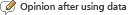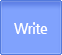연구성과물검색
유형별/분류별 연구성과물 검색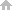HOME > Search by Achievements Type > Reports View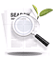Reports Detailed Information

Dirac Laplacian에 대한 relative zeta-determinant와 scattering 이론
Reports NRF is supported by Research Projects( Dirac Laplacian에 대한 relative zeta-determinant와 scattering 이론 | 2005 Year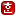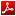| 이윤원(인하대학교)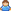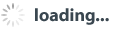) data is submitted to the NRF Project Results
Researcher who has been awarded a research grant by Humanities and Social Studies Support Program of NRF has to submit an end product within 6 months(* depend on the form of business)
• Researchers have entered the information directly to the NRF of Korea research support system
 Project Number C00008 Year(selected) 2005 Year the present condition of Project 종료 State of proposition 재단승인 Completion Date 2006년 07월 08일 Year type 결과보고 Year(final report) 2006년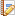Research Summary
•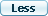Korean
• 이 과제에서 본인은 Laplacian에 대한 zeta-determinant의 gluing formula에 대한 연구를 수행하였다. Gluing formula는 주어진 다양체를 두 조각으로 쪼갰을 때, 전체 다양체에서의 zeta-determinant와 쪼개진 각각의 부분에서의 zeta-determinant와의 차이를 쪼갠 면에서의 적당한 항목으로 표현하는 공식을 말한다. Compact 다양체 위에서의 Laplacian의 zeta-determinant에 대한 gluing formula는 90년대 초반에 Burghelea, Friedlander, Kappeler에 의해서 증명이 되었고 (BFK-gluing formula), 다시 2000년대 초반에 Carron에 의해서 non-compact 다양체의 경우로 확장되었다. 그런데 이들 공식에는 알려지지 않은 상수를 하나 포함하고 있는데, 이 상수는 parameter dependent Dirichlet-to Neumann 작용소의 zeta-determinant에 대한 asymptotic expansion의 zero-coefficient로 표현이 될 뿐이다. 짝수 차원의 다양체에서는 이 상수가 0임이 알려져 있고, 주어진 다양체를 자르는 hypersurface 근방 (collar neighborhood)에서 product structure가 주어졌을 때에는 이 상수가 hypersurface에서의 Laplacian에 대한 zeta 함수의 0에서의 값과 같음이 알려져 있다. Hypersurface의 collar neighborhood에서 가장 간단한 metric이 product metric이라면 다음으로 간단한 metric은 warped product metric이다. 이 과제에서는 주어진 다양체의 hypersurface 근방에 warped product metric이 주어졌을 때, BFK-gluing formula에 나타나는 상수에 대한 연구를 수행하였다. BFK-gluing formula에서는 hypersurface에서의 error term을 기술하는데 Drichlet-to-Neumann 작용소를 사용한다. 이 과제에서는, 우선 hypersurface 근방에 warped product metric이 주어졌을 때 Laplacian에 대한 parameter dependent Drichlet-to-Neumann 작용소를 보다 유용한 형태로 기술하였다. 이를 이용하여 이 작용소에 대한 zeta-determinant의 asymptotic expansion을 고찰하였다. 그 결과로 연구 목표가 되는 상수에 대한 표현식을 얻었다. 그리고 특별히 주어진 다양체의 차원이 3과 5일 때에는 이 상수를 구체적으로 계산하였다. 마지막으로, BFK-gluing formula는 relative zeta 함수의 0에서의 값에 대한 정보를 포함하고 있는데, 이 경우에 상응하는 0에서의 relative zeta 함수 값도 구하였다.
•English
• In this research subject, we study the gluing formula for the zeta-determinants of Laplacians. The gluing formula means the following. If we are given a complete Riemannian manifold, we cut this manifold along a hypersurface. Then the gluing formula for zeta-determinants of Laplacians describes the difference of the zeta-determinant on the whole manifold and the sum of the zeta-determinant in each part. The gluing formula for zeta-determinants of Laplacians was proved in the early 90's by Burghelea, Friedlander and Kappeler on a compact manifold with respect to the Dirichlet boundary condition. About 10 years later, Carron extended this result to the case of the relative zeta-determinant on a complete non-compact manifold. However, each of this formula contains an unknown constant which is expressed only by the zero coefficient in the asymptotic expansion of log of the zeta-determinants of a one-parameter family of Dirichlet-to-Neumann operators. If the dimension of a manifold is even, this constant is known to be zero. Hence the research should be focused on an odd dimensional manifold. If a manifold has the product structure (the product metric and the corresponding Laplacian) near the cutting hypersurface, this constant is equal to the value of the zeta function at zero for the Laplacian on the cutting hypersurface. Certainly the simpliest metric on a collar neighborhood of a cutting hypersurface is the product metric and hence it is natural to discuss this constant when the warped product metric is given on the collar neighborhood of a cutting hypersurface, which is the main purpose of this research subject.
The Dirichlet-to-Neumann operator plays a central role in the BFK-gluing formula but this operator is not easy to describe and to control. When the warped product metric is given on a collar neighborhood of a cutting hypersurface, we first describe the one-parameter family of Dirichlet-to-Neumann operators into a more useful form. Using this result, we investigate the asymptotic expansion of log of the zeta-determinants of the one-parameter family of Dirichlet-to-Neumann operators, specially the zero coefficient of this asymptotic expansion. As a result we obtain a formal expression for this constant and compute this constant explicitly when the dimension of a manifold is 3 and 5.
On the other hand, the BFK-gluing formula gives some informations about the values of relative zeta functions at zero. As a byproduct, we compute the values relative zeta functions at zero for Laplacians and give two applications for this result. We first compute the value of the zeta function at zero for a compatible Dirac Laplacian with the Atiyah-Patodi-Singer (APS)boundary condition on a compact manifold with boundary when the product metric is given near the boundary. Next, we compute the value of the relative zeta function at zero for a compatible Dirac Laplacian on a manifold with cylindrical end when the Dirichlet or the APS boundary condition is imposed on the bottom of the cylinder. At this point the scattering theory is involved.
Finally, by a simple observation, we show that the constant in the BFK-gluing formula is determined completely by the data of a collar neighborhood of the cutting hypersurface and the Laplacian on this collar neighborhood.Research result report
•Abstract
• The zeta-determinants or the relative zeta-determinants of Laplacians are global spectral invariants of elliptic operators on complete Riemannian manifolds. In case of global invariants, it is natural to discuss the gluing formula in the same line with the Seifert van Kampen Theorem or the Mayer-Vietoris sequence. In case of the zeta-determinants of elliptic operators, the gluing formula was proved by Burghelea, Friedlander and Kappeler (BFK-gluing formula) in 1992 on a compact manifold with respect to the Dirichlet boundary condition. In 2002, Carron extended this result to the case of a complete non-compact manifold. But their formulas contain unknown constants which are expressed only by the zero coefficients of some asymptotic expansions. If the dimension of a manifold is even, this constant is known to be zero. Hence the research should be focused on an odd-dimensional manifold. If a manifold has the product structure on a collar neighborhood of a cutting hypersurface, this constant was computed explicitly by Carron and the author. In this subject we discuss this constant when the warped product metric is given on a collar neighborhood of a cutting hypersurface.
The Dirichlet-to-Neumann operator defined on the cutting hypersurface plays a central role in the BFK-gluing formula. In fact, this constant is the constant term in the asymptotic expansion of log of zeta-determinants of a one-parameter family of the Dirichlet-to-Neumann operators. But usually the Dirichlet-to-Neumann operator is not easy to describe or to control. In this subject, when the warped product metric is given near the cutting hypersurface, we first describe the Dirichlet-to-Neumann operator into a more useful form and discuss the asymptotic expansion of log of zeta-determinants with focusing on the constant term. As a result, we obtain a formal expression for this constant in terms of the short time asymptotics of the heat kernel of the Dirichlet-to-Neumann operator. Moreover, we compute this constant explicitly when the dimension of a manifold is 3 and 5. As a simple observation, we show that this constant is determined by the data of the manifold and the Laplacian on a collar neighborhood of the cutting hypersurface, not by the data of the cutting hypersurface itself and the Laplacian restricted on the cutting hypersurface.
On the other hand, the BFK-gluing formula contains some informations about the values of relative zeta functions at zero. As a byproduct we compute the values of relative zeta functions at zero when the warped product metric is given on a collar neighborhood of a cutting hypersurface. We give two applications for this result. Using this result, we first compute the values of zeta functions at zero for Dirac Laplacians with the Atiyah-Patodi-Singer (APS) boundary condition on a compact manifold with boundary when the product metric is given neat the boundary. Next, we compute the values of the relative zeta functions for Laplacians at zero on a manifold with cylindrical ends when the Dirichlet or the APS boundary condition is imposed on the bottom of the cylinder. At this point, the scattering theory is involved.
•Research result and Utilization method
• To complete the BFK-gluing formula for the (relative) zeta-determinants of Laplacians we need to understand this constant explicitly. What is known until now is the following. If the dimension of a manifold is even, this constant is zero. If a manifold has the product structure (the product metric and the corresponding Laplacian), this constant is equal to the value of the zeta function at zero for the Laplacian restricted to the cutting hypersurface. In these two cases, this constant is determined completely by the data of the cutting hypersurface. In this subject, by considering more complicate case (the case of warped product metric), we show that this constant is not determined only by the data of the cutting hypersurface itself. We show how this constant depends on the data of a collar neighborhood of the cutting hypersurface.
• Index terms
• (relative) zeta-determinant, BFK-gluing formula, Dirichlet-to-Neumann operator, Dirichlet boundary condition, warped product metric.
•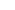List of digital content of this reports• This document, it is necessary to display the original author and you do not have permission